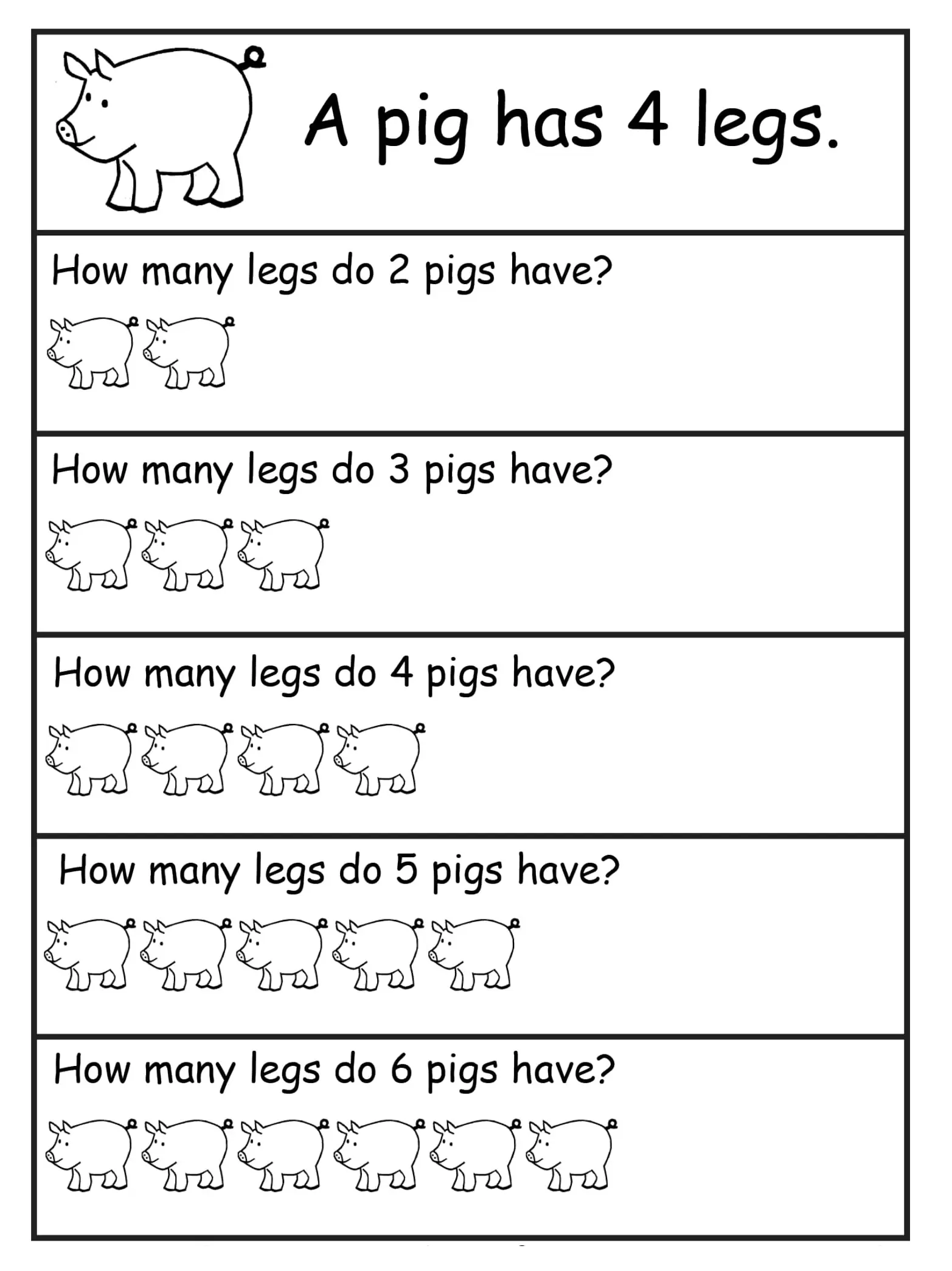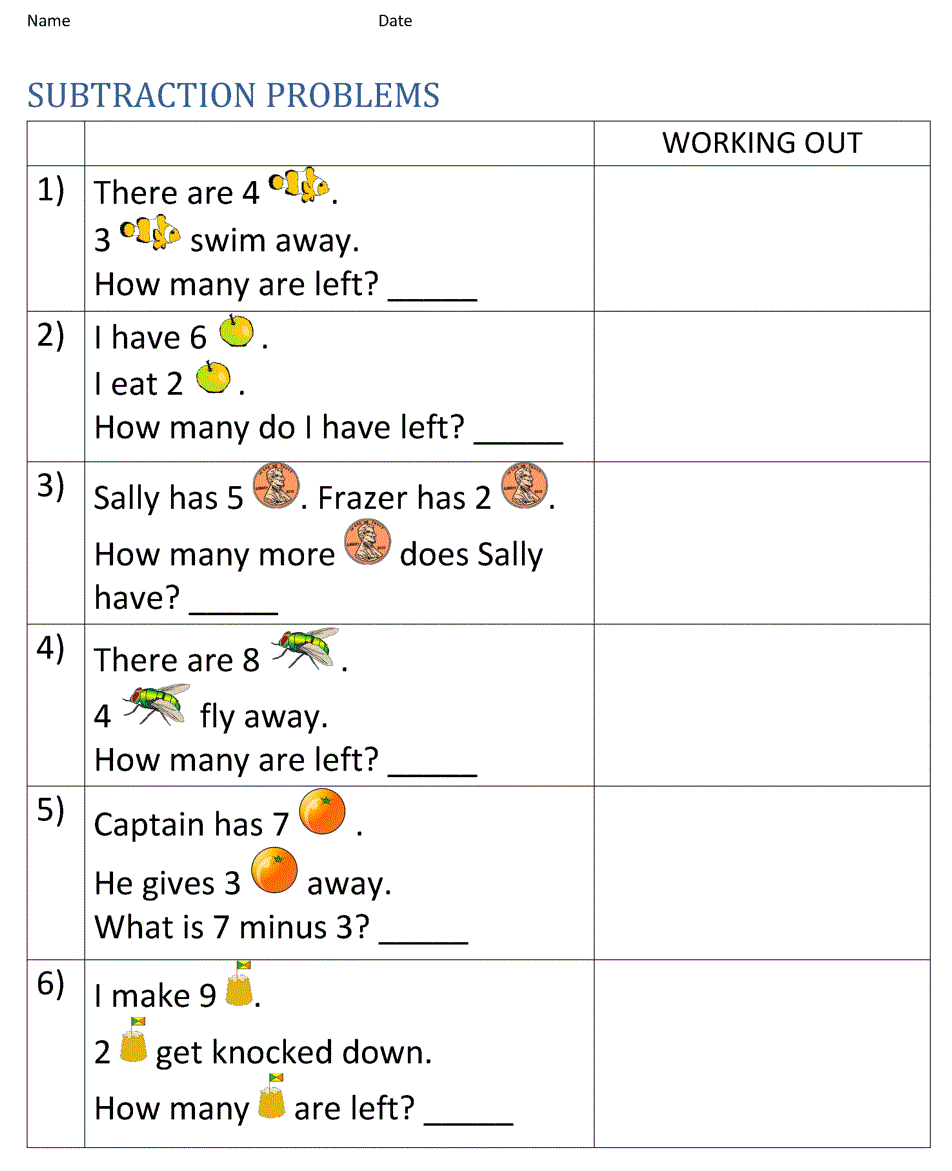# 10 Amazing 1st Grade Math Word Problems Worksheets Samples

Children in grade 1 will learn about mathematics for the first time. Grade 1 students will be taught addition, subtraction, division, pattern numbers, comparisons, and other basic math. To help students improve their math skills , teachers usually use 1st grade math word problems worksheets. The worksheets contain short stories, in the form of word problems about a mathematical problem. For example, “Andrew has 7 apples and Ana has 3 apples. How many apples did Andrew and Ana have?”

We provide the following first grade math word problems worksheets to help teacher teaching their students. Our math worksheet available in printable format: JPG, PDF, and Docx.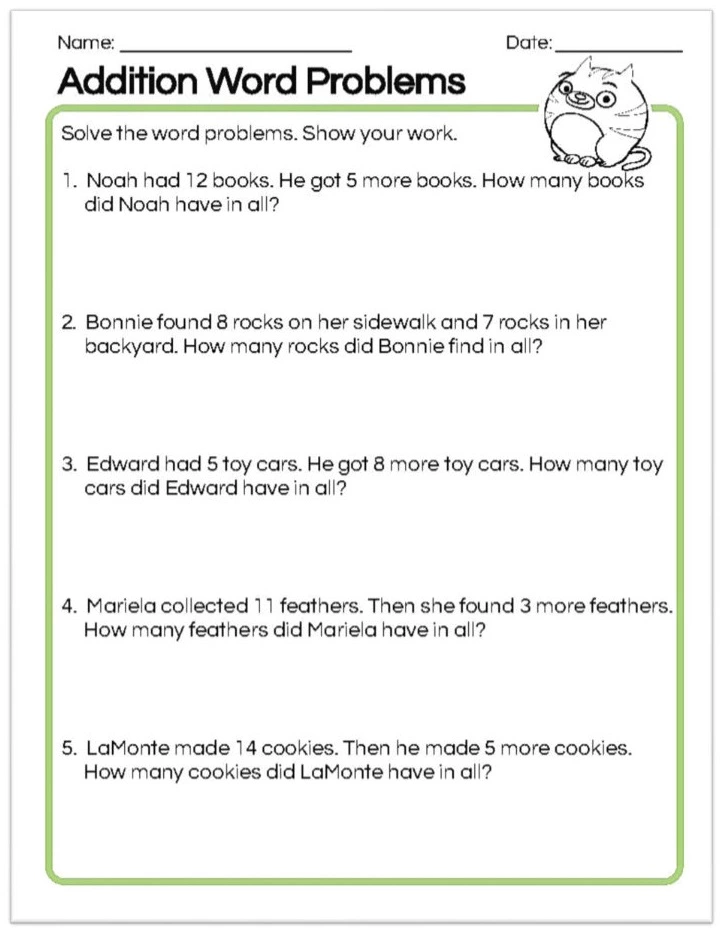This worksheet contains 5 questions of addition word problems for 1st-grade students.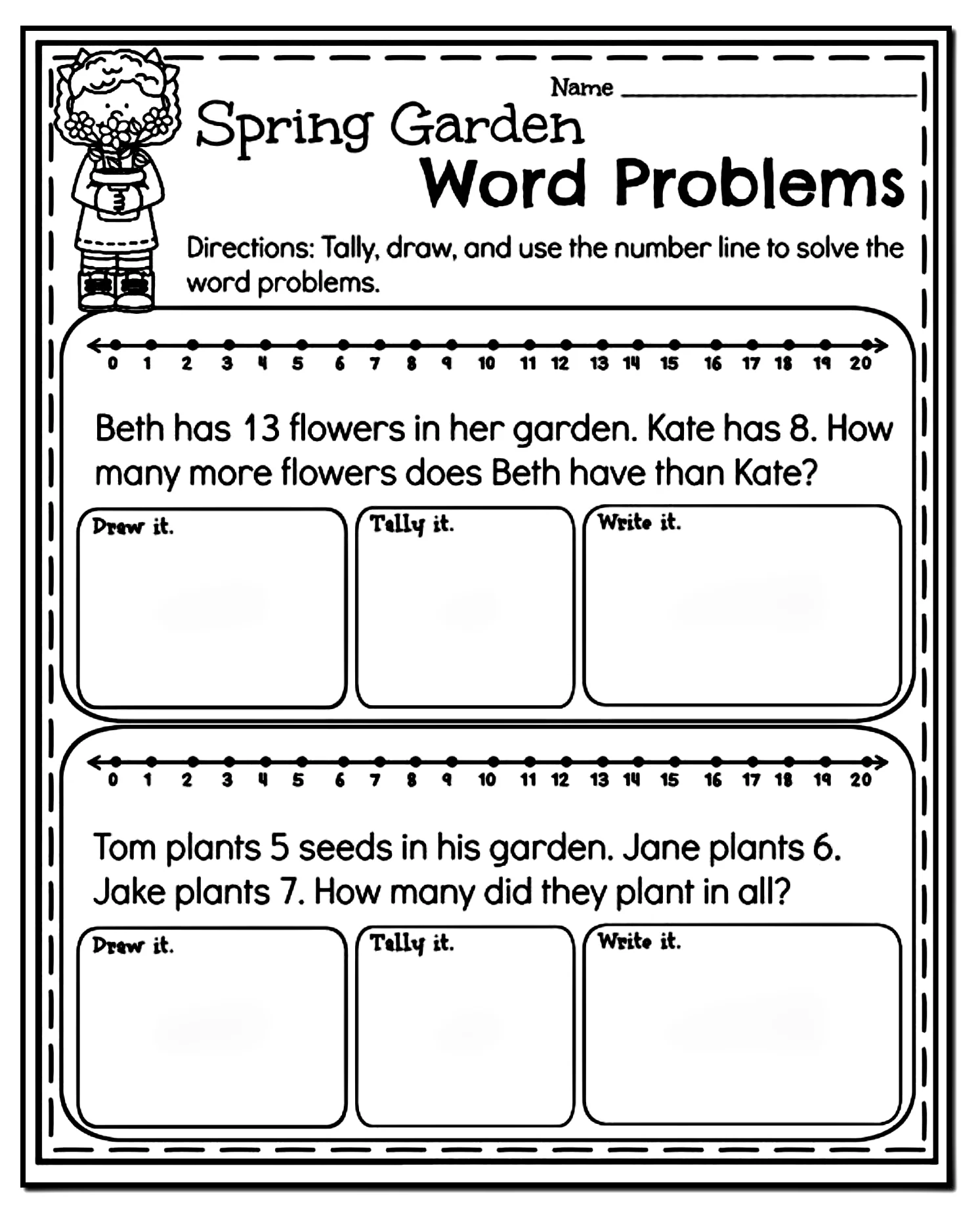Spring garden word worksheet is good worksheet for student to learn to draw, count, and writing number.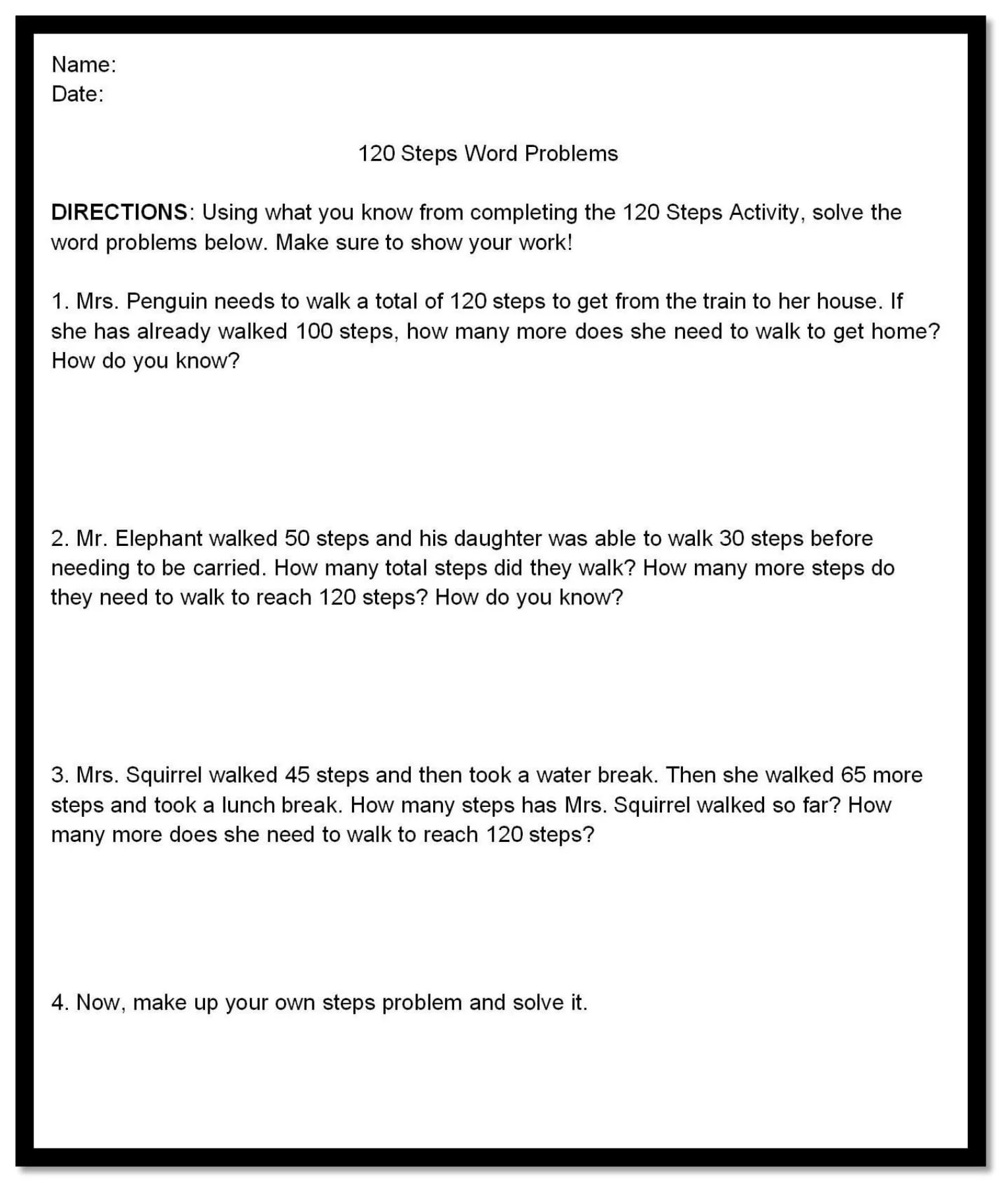Students can practice the questions on this worksheet in class, by following the instructions.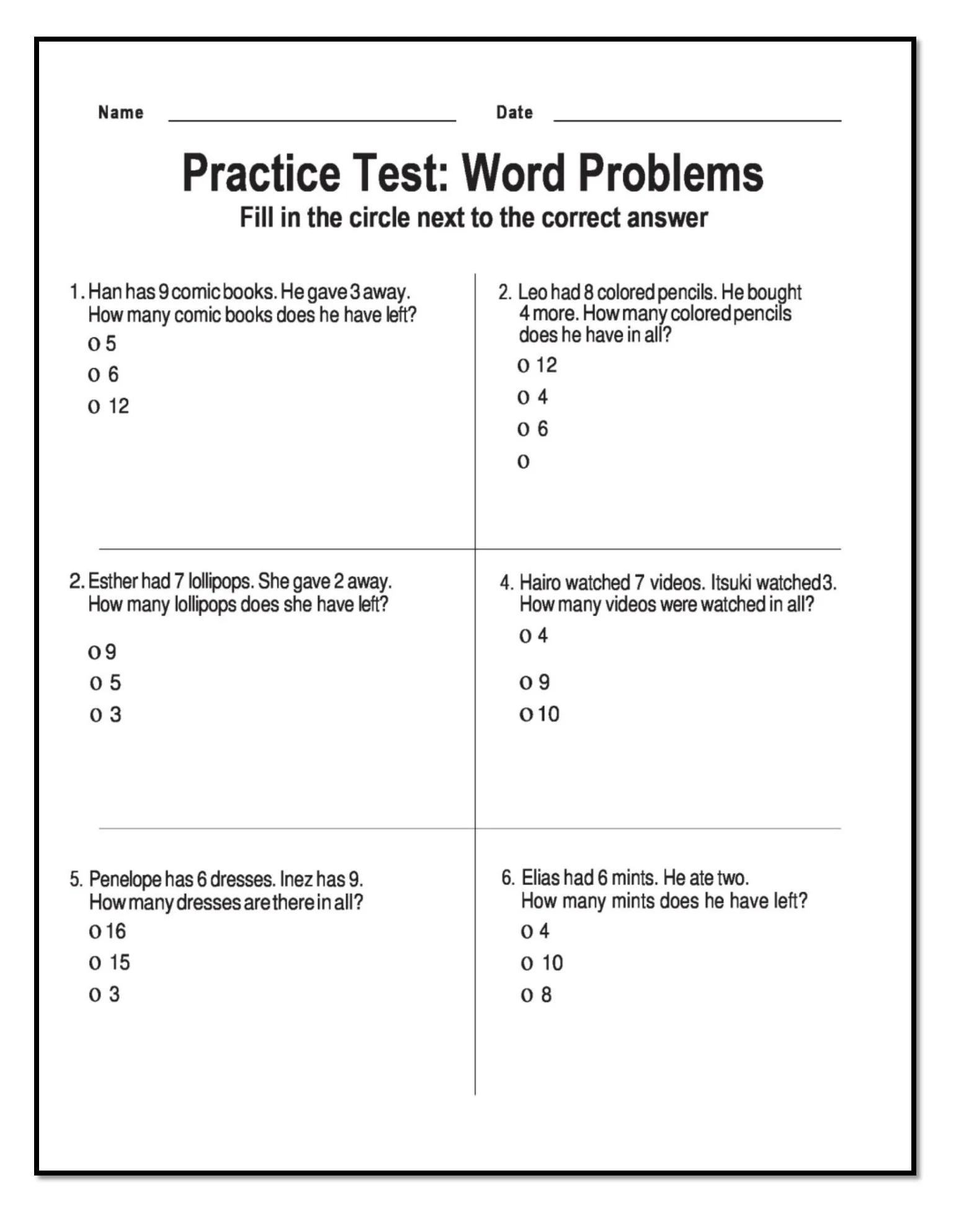Combination of addition and subtraction in one worksheet for practicing solve math problems.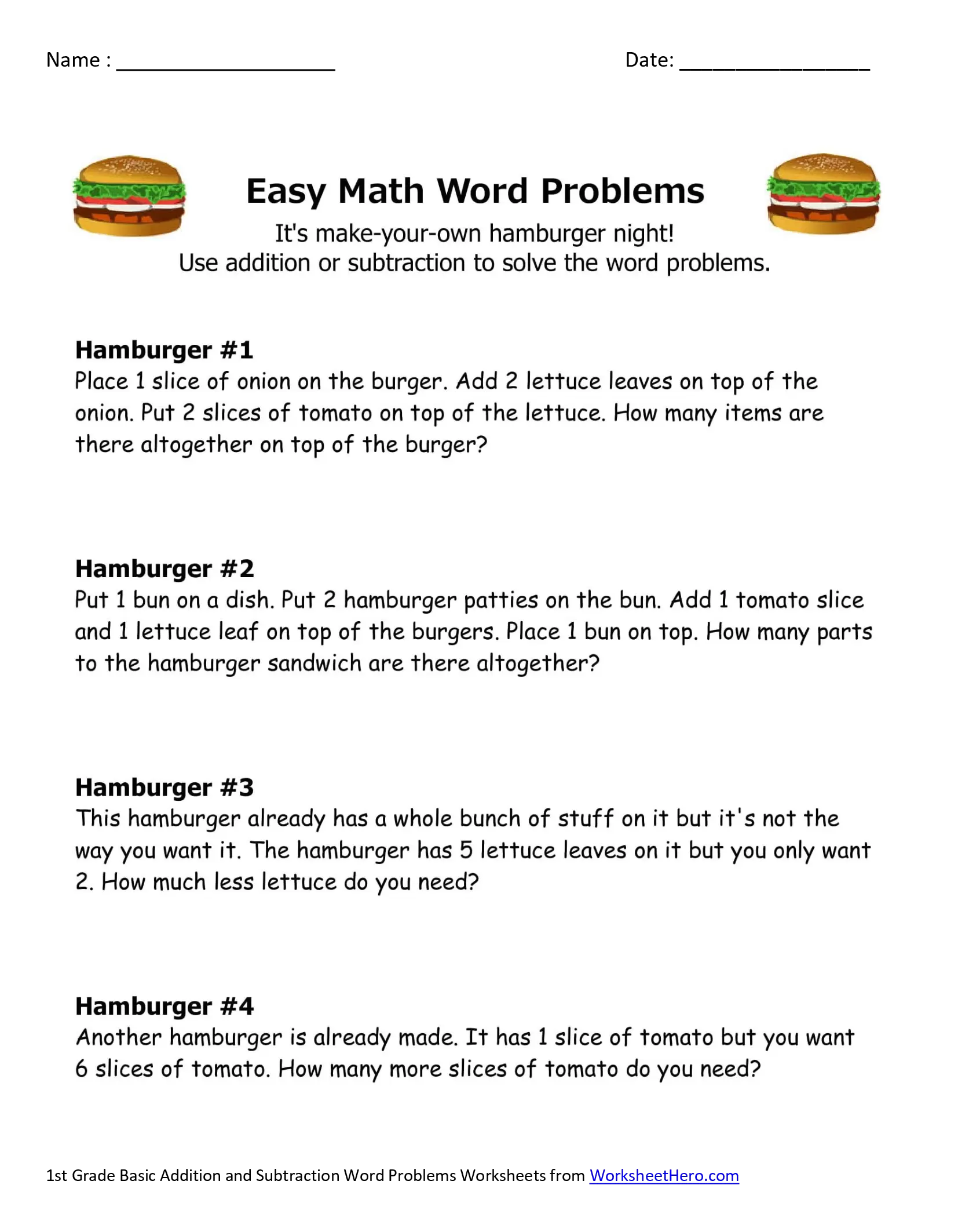This 1st grade math word problem worksheets  example of using real live problem to solve first grade math problems. Hamburger is a familiar food for the student, so they can imagine it to help them answer math question in this worksheet.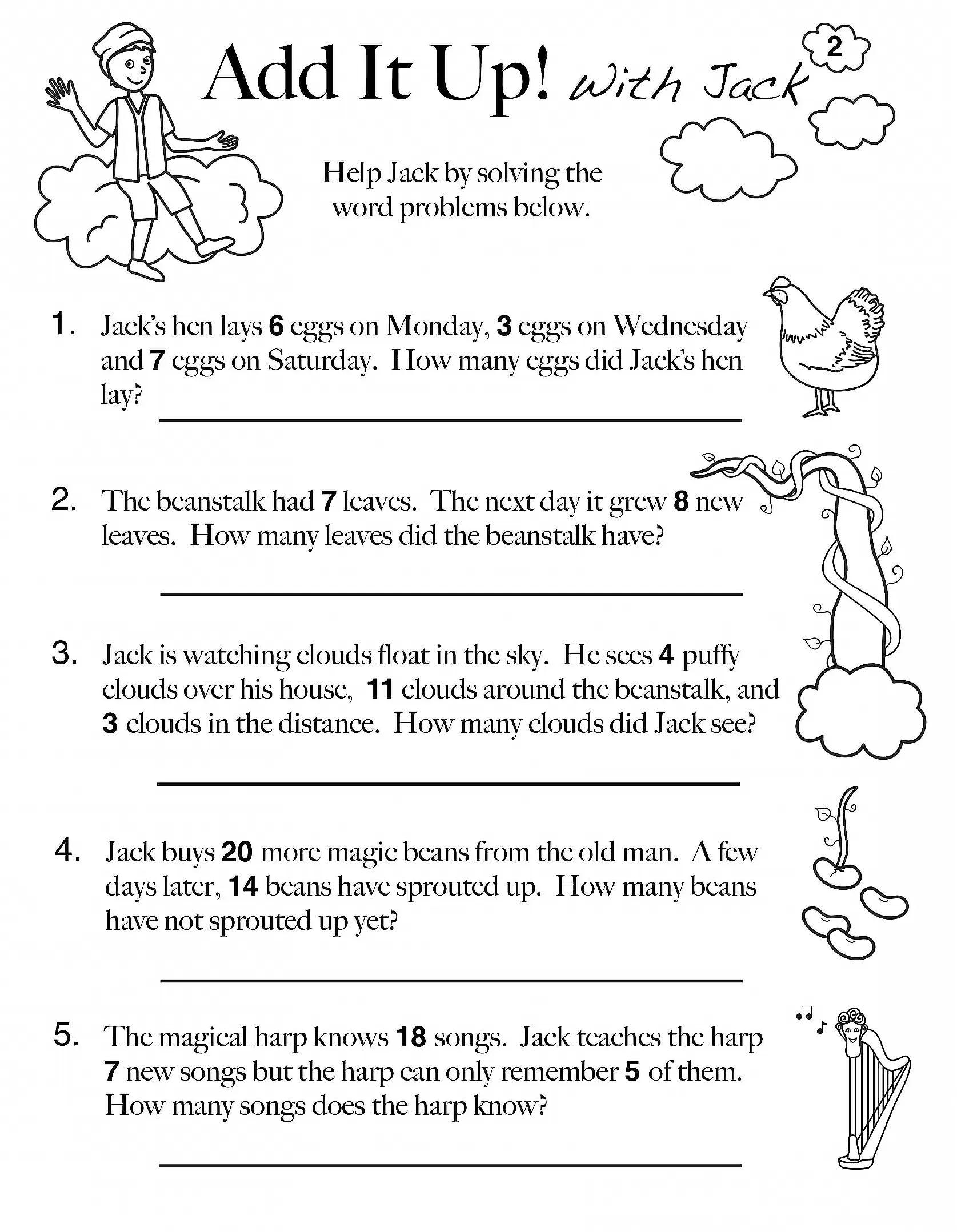This math word worksheet use Jack and the Beanstalk story as an illustration. Students ask to help Jack solving word problems.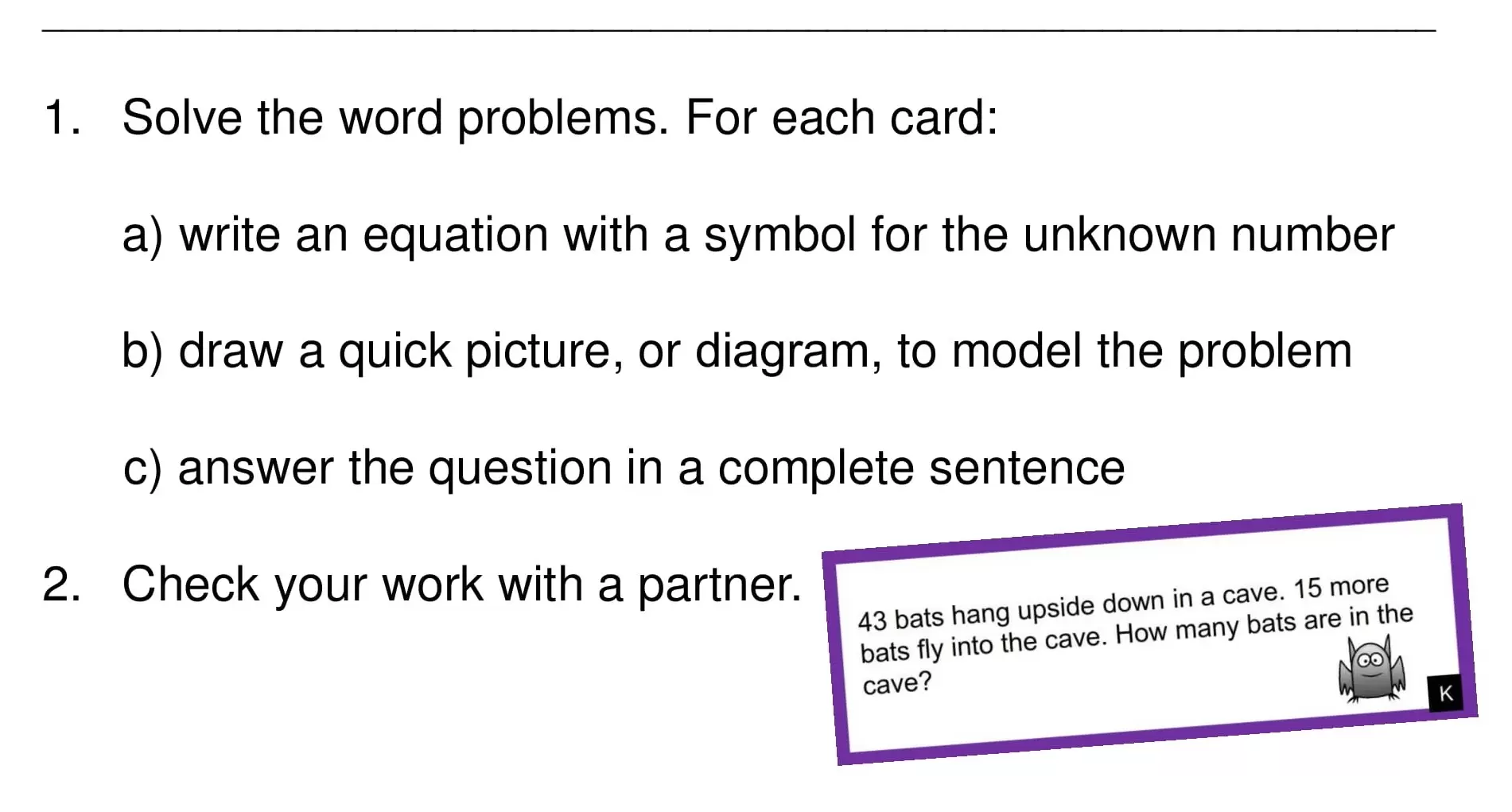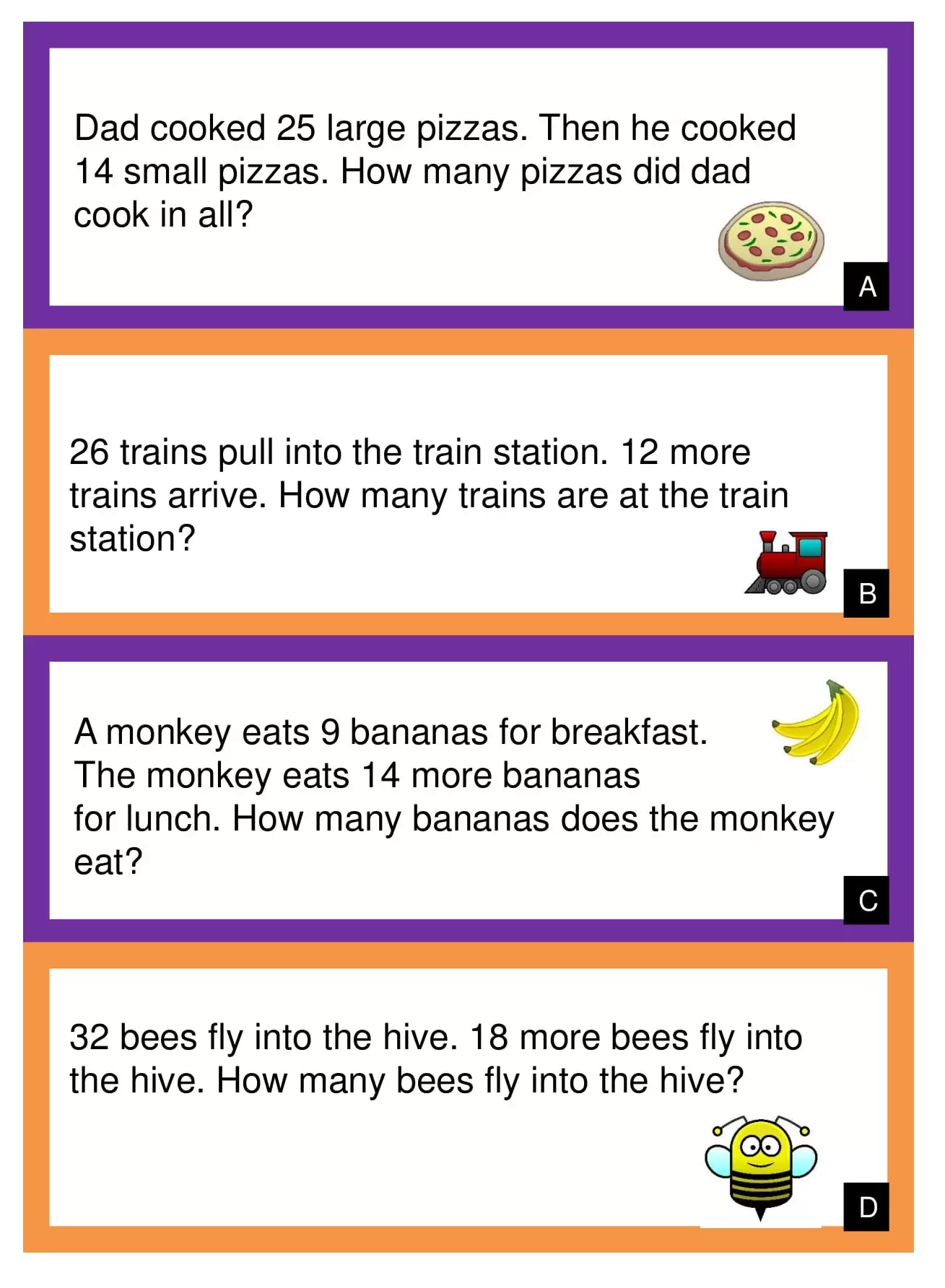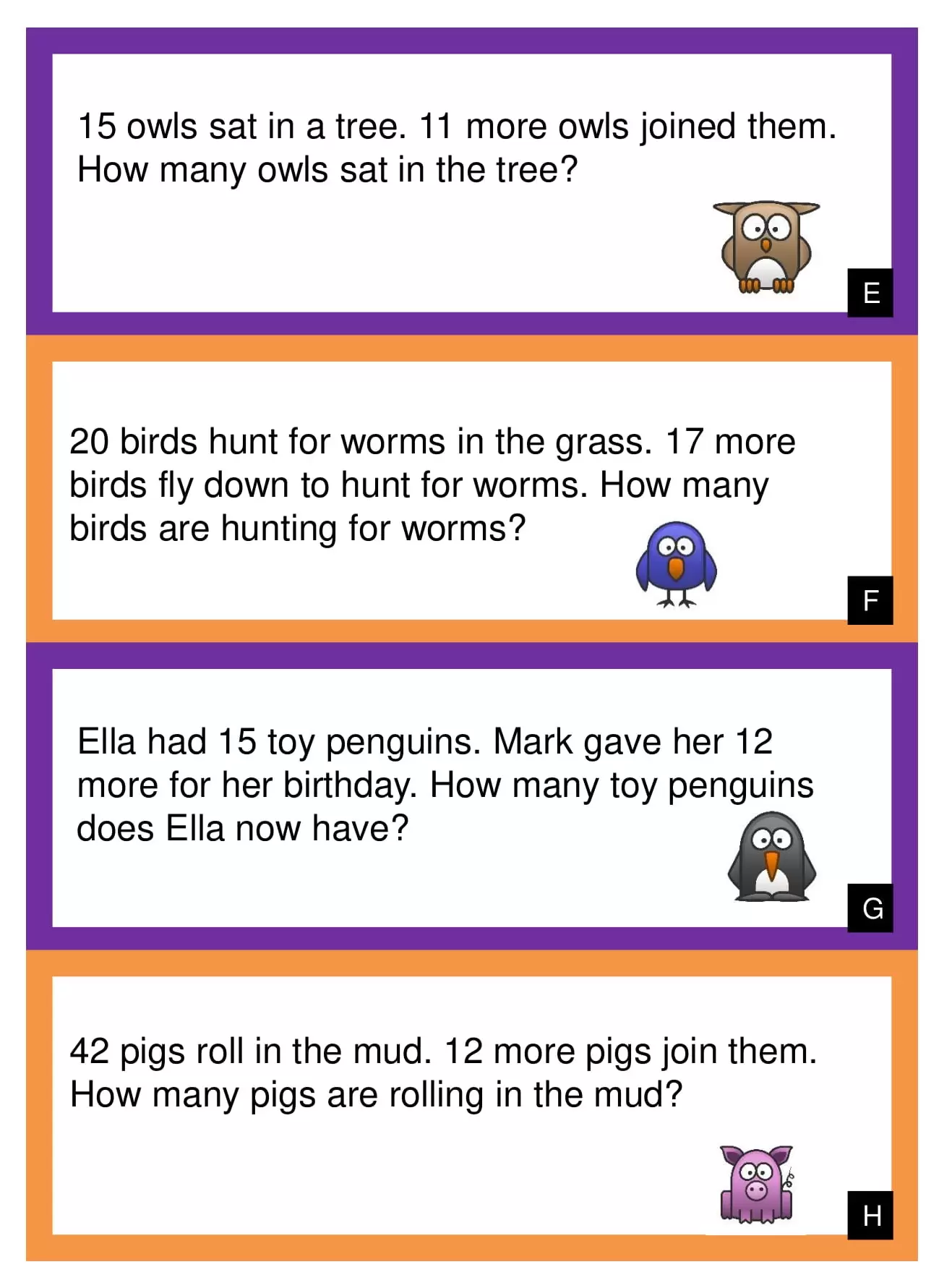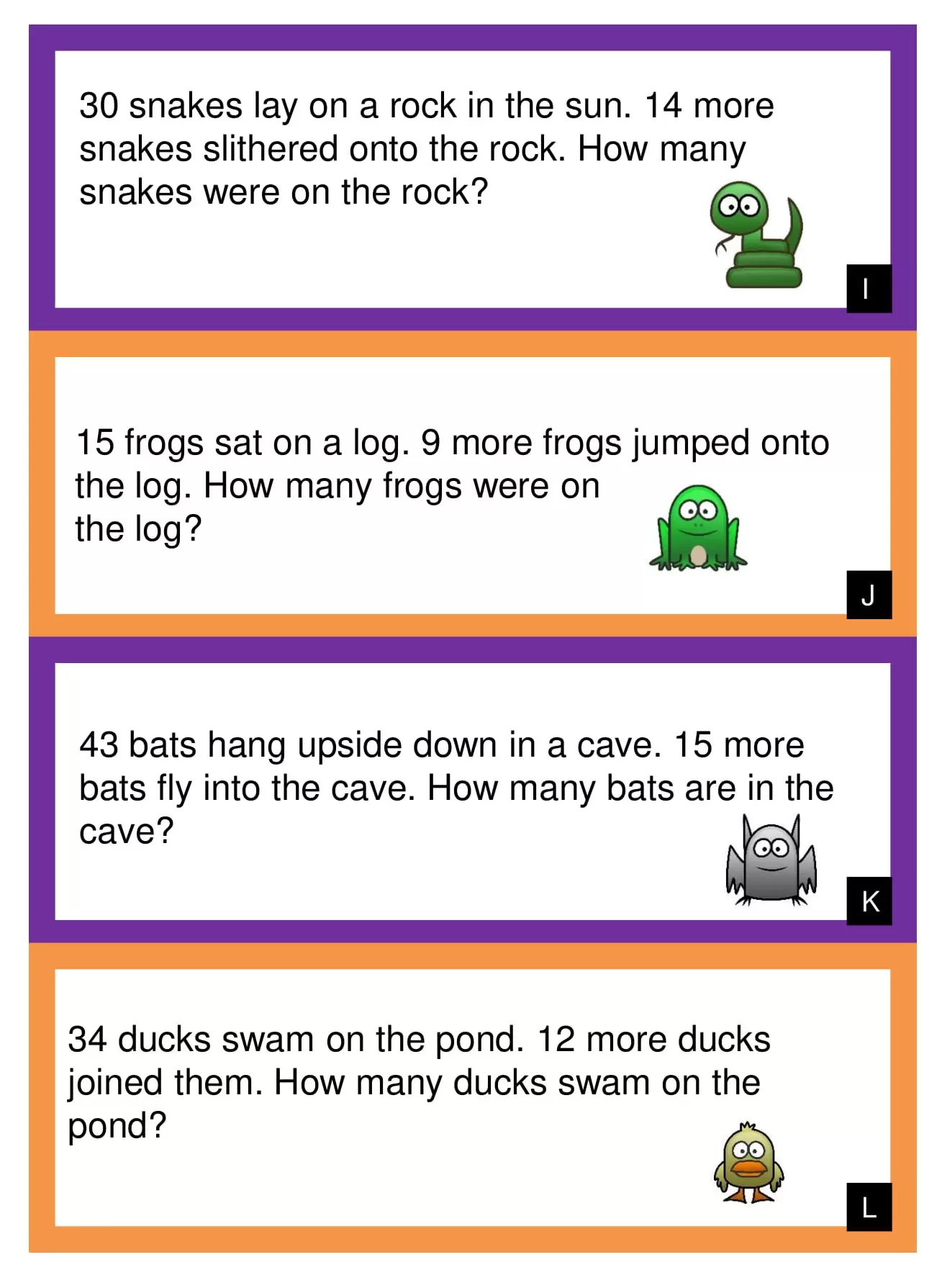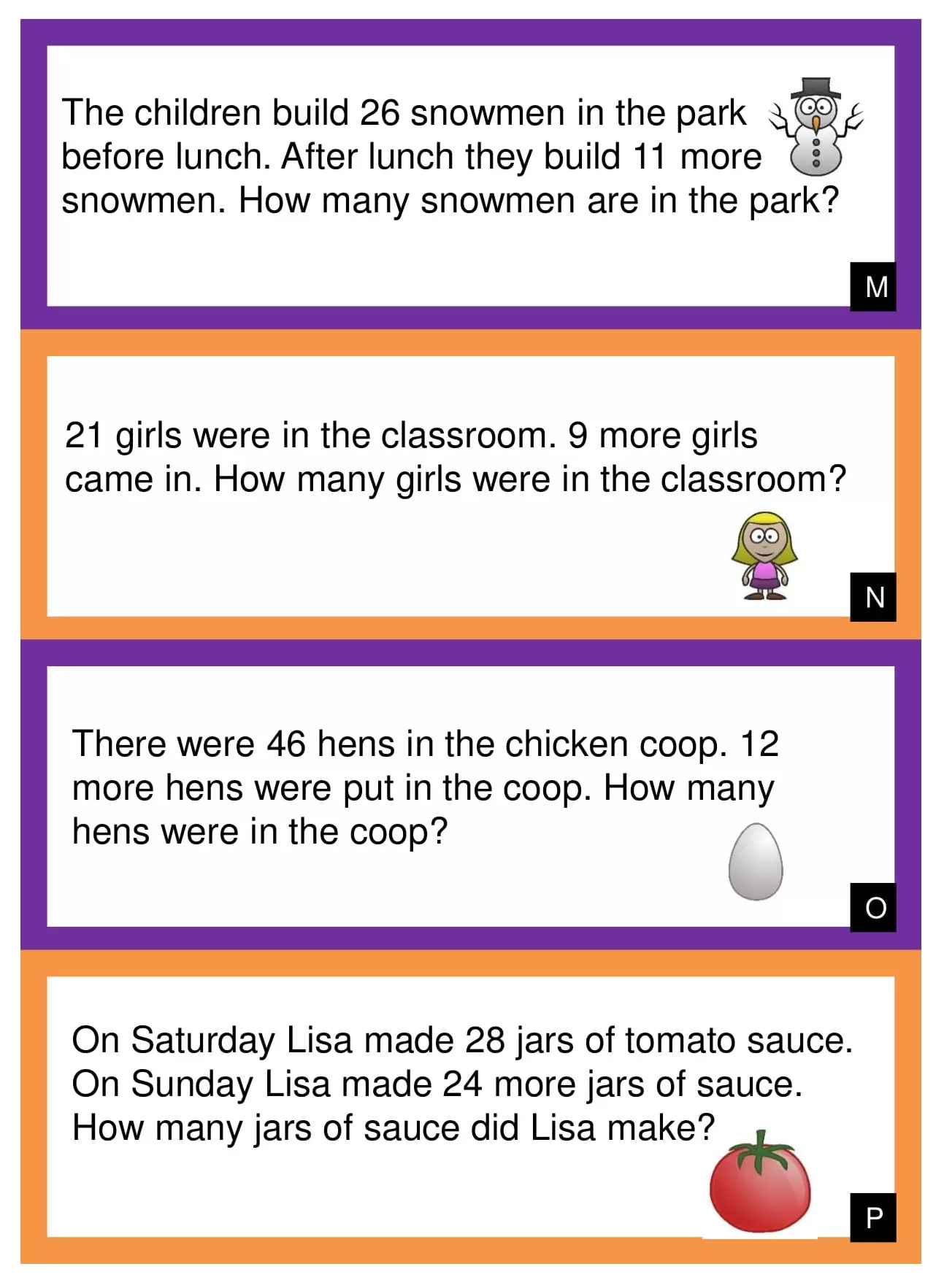## 1st Grade Multiplication Word Problems Worksheets